###### Introduction

Quad trees are hierarchical spatial tree data structures that are based on the principle of recursive decomposition of space. The term quad tree originated from representation of two dimensional data by recursive decomposition of space using separators parallel to the co- ordinate axis. The resulting split of a region into four regions corresponding to southwest, northwest, southeast and northeast quadrants is represented as four children of the node corresponding to the region, hence the term“quad”tree. In a three dimensional analogue, a region is split into eight regions using planes parallel to the coordinate planes. As each internal node can have eight children corresponding to the 8-way split of the region associated with it, the term octree is used to describe the resulting tree structure. Analogous data structures for representing spatial data in higher than three dimensions are called hyperoc trees. It is also common practice to use the term quad trees in a generic way irrespective of the dimensionality of the spatial data. This is especially useful when describing algorithms that are applicable regardless of the speciﬁc dimensionality of the underlying data.

Several related spatial data structures are described under the common rubric of quadtrees. Common to these data structures is the representation of spatial data at various levels of granularity using a hierarchy of regular, geometrically similar regions (such as cubes, hy- perrectangles etc.). The tree structure allows quick focusing on regions of interest, which facilitates the design of fast algorithms. As an example, consider the problem of ﬁnding all points in a data set that lie within a given distance from a query point, commonly known as the spherical region query. In the absence of any data organization, this requires checking the distance from the query point to each point in the data set. If a quadtree of the data is available, large regions that lie outside the spherical region of interest can be quickly discarded from consideration, resulting in great savings in execution time. Furthermore, the unit aspect ratio employed in most quad tree data structures allows geometric arguments useful in designing fast algorithms for certain classes of applications.

In constructing a quad tree, one starts with a square, cubic or hypercubic region (depending on the dimensionality) that encloses the spatial data under consideration. The diﬀerent variants of the quad tree data structure are diﬀerentiated by the principle used in the re- cursive decomposition process. One important aspect of the decomposition process is if the decomposition is guided by input data or is based on the principle of equal subdivision of the space itself. The former results in a tree size proportional to the size of the input. If all the input data is available a priori, it is possible to make the data structure height balanced. These attractive properties come at the expense of diﬃculty in making the data structure dynamic, typically in accommodating deletion of data. If the decomposition is based on equal subdivision of space, the resulting tree depends on the distribution of spatial data. As a result, the tree is height balanced and is linear in the size of input only when the distribution of the spatial data is uniform, and the height and size properties deteriorate with increase in nonuniformity of the distribution. The beneﬁcial aspect is that the tree structure facilitates easy update operations and the regularity in the hierarchical representation of the regions facilitates geometric arguments helpful in designing algorithms.

Another important aspect of the decomposition process is the termination condition to stop the subdivision process. This identiﬁes regions that will not be subdivided further, which will be represented by leaves in the quadtree. Quadtrees have been used as ﬁxed res- olution data structures, where the decomposition stops when a preset resolution is reached, or as variable resolution data structures, where the decomposition stops when a property based on input data present in the region is satisﬁed. They are also used in a hybrid man- ner, where the decomposition is stopped when either a resolution level is reached or when a property is satisﬁed.

Quadtrees are used to represent many types of spatial data including points, line segments, rectangles, polygons, curvilinear objects, surfaces, volumes and cartographic data. Their use is pervasive spanning many application areas including computational geometry, computer aided design (Chapter 52), computer graphics (Chapter 54), databases (Chap- ter 60), geographic information systems (Chapter 55), image processing (Chapter 57), pat- tern recognition, robotics and scientiﬁc computing. Introduction of the quad tree data structure and its use in applications involving spatial data dates back to the early 1970s and can be attributed to the work of Klinger , Finkel and Bentley , and Hunter . Due to extensive research over the last three decades, a large body of literature is available on quad trees and its myriad applications. For a detailed study on this topic, the reader is referred to the classic textbooks by Samet [29, 30]. Development of quad tree like data structures, algorithms and applications continues to be an active research area with signiﬁ- cant research developments in recent years. In this chapter, we attempt a coverage of some of the classical results together with some of the more recent developments in the design and analysis of algorithms using quad trees and octrees.

## Quad trees for Point Data

We ﬁrst explore quad trees in the context of the simplest type of spatial data multidimensional points. Consider a set of n points in d dimensional space. The principal reason a spatial data structure is used to organize multidimensional data is to facilitate queries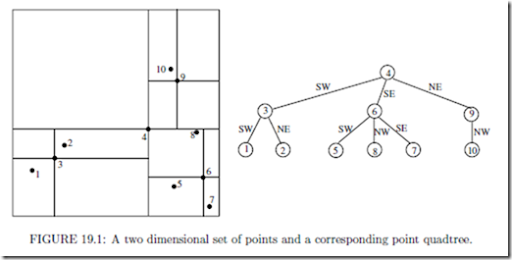requiring spatial information. A number of such queries can be identiﬁed for point data. For example:

1. Range query: Given a range of values for each dimension, ﬁnd all the points that lie within the range. This is equivalent to retrieving the input points that lie within a speciﬁed hyper rectangular region. Such a query is often useful in database information retrieval.

2. Spherical region query: Given a query point p and a radius r, ﬁnd all the points that lie within a distance of r from p. In a typical molecular dynamics application, spherical region queries centered around each of the input points is required.

3. All nearest neighbor query: Given n points, ﬁnd the nearest neighbor of each point within the input set.

While quad trees are used for eﬃcient execution of such spatial queries, one must also design algorithms for the operations required of almost any data structure such as constructing the data structure itself, and accommodating searches, insertions and deletions. Though such algorithms will be covered ﬁrst, it should be kept in mind that the motivation behind the data structure is its use in spatial queries. If all that were required was search, insertion and deletion operations, any one dimensional organization of the data using a data structure such as a binary search tree would be suﬃcient.

The point quad tree is a natural generalization of the binary search tree data structure to multiple dimensions. For convenience, ﬁrst consider the two dimensional case. Start with a square region that contains all of the input points. Each node in the point quad tree corresponds to an input point. To construct the tree, pick an arbitrary point and make it the root of the tree. Using lines parallel to the coordinate axis that intersect at the selected point (see Figure 19.1), divide the region into four subregions corresponding to the southwest, northwest, southeast and northeast quadrants, respectively. Each of the subregions is recursively decomposed in a similar manner to yield the point quad tree. For points that lie at the boundary of two adjacent regions, a convention can be adopted to treat the points as belonging to one of the regions. For instance, points lying on the left and bottom edges of a region may be considered included in the region, while points lying on the top and right edges are not. When a region corresponding to a node in the tree contains a single point, it is considered a leaf node. Note that point quad trees are not unique and their structure depends on the selection of points used in region subdivisions. Irrespective of the choices made, the resulting tree will have n nodes, one corresponding to each input point.

If all the input points are known in advance, it is easy to choose the points for subdivision so as to construct a height balanced tree. A simple way to do this is to sort the points with one of the coordinates, say x, as the primary key and the other coordinate, say y, as the secondary key. The ﬁrst subdivision point is chosen to be the median of this sorted data. This will ensure that none of the children of the root node receives more than half the points. In O(n) time, such a sorted list can be created for each of the four resulting subregions. As the total work at every level of the tree is bounded by O(n), and there are at most O(log n) levels in the tree, a height balanced point quad tree can be built in O(n log n) time. Generalization to d dimensions is immediate, with O(dn log n) run time.

The recursive structure of a point quad tree immediately suggests an algorithm for search- ing. To search for a point, compare it with the point stored at the root. If they are diﬀerent, the comparison immediately suggests the subregion containing the point. The search is directed to the corresponding child of the root node. Thus, search follows a path in the quad tree until either the point is discovered, or a leaf node is reached. The run time is bounded by O(dh), where h is the height of the tree.

To insert a new point not already in the tree, ﬁrst conduct a search for it which ends in a leaf node. The leaf node now corresponds to a region containing two points. One of them is chosen for subdividing the region and the other is inserted as a child of the node corresponding to the subregion it falls in. The run time for point insertion is also bounded by O(dh), where h is the height of the tree after insertion. One can also construct the tree itself by repeated insertions using this procedure. Similar to binary search trees, the run time under a random sequence of insertions is expected to be O(n log n) . Overmars and van Leeuwen  present algorithms for constructing and maintaining optimized point quad trees irrespective of the order of insertions.

Deletion in point quad trees is much more complex. The point to be deleted is easily identiﬁed by a search for it. The diﬃculty lies in identifying a point in its subtree to take the place of the deleted point. This may require nontrivial readjustments in the subtree underneath. The reader interested in deletion in point quadtrees is referred to . An analysis of the expected cost of various types of searches in point quad trees is presented by Flajolet et al. .

For the remainder of the chapter, we will focus on quad tree data structures that use equal subdivision of the underlying space, called region quad trees. This is because we regard Bentley’s multidimensional binary search trees , also called kd trees, to be superior to point quad trees. The kd tree is a binary tree where a region is subdivided into two based only on one of the dimensions. If the dimension used for subdivision is cyclically rotated at consecutive levels in the tree, and the subdivision is chosen to be consistent with the point quad tree, then the resulting tree would be equivalent to the point quad tree but without the drawback of large degree (2d in d dimensions). Thus, it can be argued that point quad trees are contained in kd trees. Furthermore, recent results on compressed region quad trees indicate that it is possible to simultaneously achieve the advantages of both region and point quad trees. In fact, region quad trees are the most widely used form of quad trees despite their dependence on the spatial distribution of the underlying data. While their use posed theoretical inconvenience — it is possible to create as large a worst-case tree as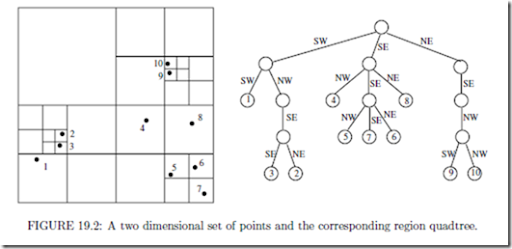desired with as little as three points — they are widely acknowledged as the data structure of choice for practical applications. We will outline some of these recent developments and outline how good practical performance and theoretical performance guarantees can both be achieved using region quad trees.

The region quad tree for n points in d dimensions is deﬁned as follows: Consider a hypercube large enough to enclose all the points. This region is represented by the root of the d– dimensional quadtree. The region is subdivided into 2d subregions of equal size by bisecting along each dimension. Each of these regions containing at least one point is represented as a child of the root node. The same procedure is recursively applied to each child of the root node. The process is terminated when a region contains only a single point. This data structure is also known as the point region quad tree, or PR-quad tree for short . At times, we will simply use the term quad tree when the tree implied is clear from the context. The region quad tree corresponding to a two dimensional set of points is shown in Figure 19.2. Once the enclosing cube is speciﬁed, the region quad tree is unique. The manner in which a region is subdivided is independent of the speciﬁc location of the points within the region. This makes the size of the quad tree sensitive to the spatial distribution of the points.

Before proceeding further, it is useful to establish a terminology to describe the type of regions that correspond to nodes in the quad tree. Call a hypercubic region containing all the points the root cell. Deﬁne a hierarchy of cells by the following: The root cell is in the hierarchy. If a cell is in the hierarchy, then the 2d equal-sized cubic subregions obtained by bisecting along each dimension of the cell are also called cells and belong to the hierarchy (see Figure 19.3 for an illustration of the cell hierarchy in two dimensions). We use the term subcell to describe a cell that is completely contained in another. A cell containing the subcell is called a supercell. The subcells obtained by bisecting a cell along each dimension are called the immediate subcells with respect to the bisected cell. Also, a cell is the immediate supercell of any of its immediate subcells. We can treat a cell as a set of all points in space contained in the cell. Thus, we use C D to indicate that the cell C is a subcell of the cell D and C D to indicate that C is a subcell of D but C j= D.

Deﬁne the length of a cell C, denoted length(C), to be the span of C along any dimension.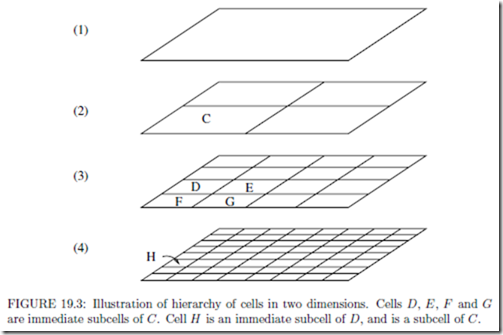An important property of the cell hierarchy is that, given two arbitrary cells, either one is completely contained in the other or they are disjoint. cells are considered disjoint if they are adjacent to each other and share a boundary.

Each node in a quad tree corresponds to a subcell of the root cell. Leaf nodes correspond to largest cells that contain a single point. There are as many leaf nodes as the number of points, n. The size of the quad tree cannot be bounded as a function of n, as it depends on the spatial distribution. For example, consider a data set of 3 points consisting of two points very close to each other and a faraway point located such that the ﬁrst subdivision of the root cell will separate the faraway point from the other two. Then, depending on the speciﬁc location and proximity of the other two points, a number of subdivisions may be necessary to separate them. In principle, the location and proximity of the two points can be adjusted to create as large a worst-case tree as desired. In practice, this is an unlikely scenario due to limits imposed by computer precision.

From this example, it is intuitively clear that a large number of recursive subdivisions may be required to separate points that are very close to each other. In the worst case, the recursive subdivision continues until the cell sizes are so small that a single cell cannot contain both the points irrespective of their location. Subdivision is never required beyond this point, but the points may be separated sooner depending on their actual location. Let s be the smallest distance between any pair of points and D be the length of the root cell. An upper bound on the height of the quad tree is obtained by considering the worst-case path needed to separate a pair of points which have the smallest pairwise distance. The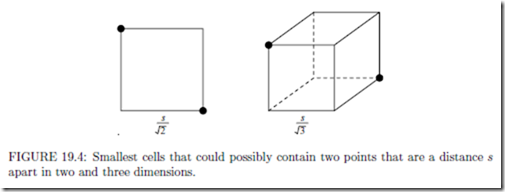worst-case path length is O(log D ). Since the tree has n leaves, the number of nodes in the tree is bounded by O(n log D ). In the worst case, D is proportional to the largest distance between any pair of points. Thus, the height of a quad tree is bounded by the logarithm of the ratio of the largest pairwise distance to the smallest pairwise distance. This ratio is a measure of the degree of nonuniformity of the distribution.

Search, insert and delete operations in region quad trees are rather straightforward. To search for a point, traverse a path from root to a leaf such that each cell on the path encloses the point. If the leaf contains the point, it is in the quad tree. Otherwise, it is not. To insert a point not already in the tree, search for the point which terminates in a leaf. The leaf node corresponds to a region which originally had one point. To insert a new point which also falls within the region, the region is subdivided as many times as necessary until the two points are separated. This may create a chain of zero or more length below the leaf node followed by a branching to separate the two points. To delete a point present in the tree, conduct a search for the point which terminates in a leaf. Delete the leaf node. If deleting the node leaves its parent with a single child, traverse the path from the leaf to the root until a node with at least two children is encountered. Delete the path below the level of the child of this node. Each of the search, insert and delete operations takes O(h) time, where h is the height of the tree. Construction of a quad tree can be done either through repeated insertions, or by constructing the tree level by level starting from the root. In either case, the worst case run time is O (n log D . We will not explore these algorithms) further in favor of superior algorithms to be described later.

In an n-leaf tree where each internal node has at least two children, the number of nodes is bounded by 2n 1. The size of quad trees is distribution dependent because there can be internal nodes with only one child. In terms of the cell hierarchy, a cell may contain all its points in a small volume so that, recursively subdividing it may result in just one of the immediate subcells containing the points for an arbitrarily large number of steps. Note that the cells represented by nodes along such a path have diﬀerent sizes but they all enclose the same points. In many applications, all these nodes essentially contain the same information as the information depends only on the points the cell contains. This prompted the development of compressed quad trees, which are obtained by compressing each such path into a single node. Therefore, each node in a compressed quad tree is either a leaf or has at least two children. The compressed quad tree corresponding to the quad tree of Figure 19.2 is depicted in Figure 19.5. Compressed quad trees originated from the work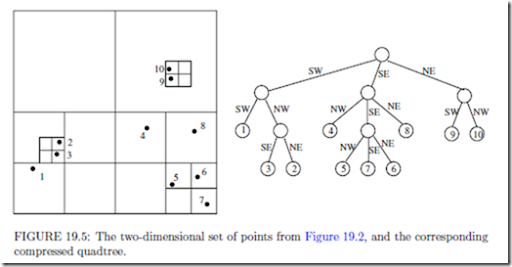of Clarkson  in the context of the all nearest neighbors problem and further studied by Aluru and Sevilgen .

A node v in the compressed quad tree is associated with two cells, large cell of v (L(v)) and small cell of v (S(v)). They are the largest and smallest cells that enclose the points in the subtree of the node, respectively. When S(v) is subdivided, it results in at least two non-empty immediate subcells. For each such subcell C resulting from the subdivision, there is a child u such that L(u) = C. Therefore, L(u) at a node u is an immediate subcell of S(v) at its parent v. A node is a leaf if it contains a single point and the small cell of a leaf node is the hypothetical cell with zero length containing the point.

The size of a compressed quad tree is bounded by O(n). The height of a compressed quad tree has a lower bound of Ω(log n) and an upper bound of O(n). Search, insert and delete operations on compressed quad trees take O(h) time. In practice, the height of a compressed quad tree is signiﬁcantly smaller than suggested by the upper bound because a) computer precision limits restrict the ratio of largest pairwise distance to smallest pairwise distance that can be represented, and b) the ratio of length scales represented by a com- pressed quadtree of height h is at least 2h : 1. In most practical applications, the height of the tree is so small that practitioners use representation schemes that allow only trees of constant height [12, 37] or even assume that the height is constant in algorithm analysis . For instance, a compressed octree of height 20 allows potentially 820 = 260 leaf nodes and a length scale of 220 : 1 106 : 1.

Though compressed quad trees are described as resulting from collapsing chains in quad trees, such a procedure is not intended for compressed quad tree construction. Instead, algorithms for direct construction of compressed quad trees in O(dn log n) time will be presented, which can be used to construct quad trees eﬃciently if necessary. To obtain a quad tree from its compressed version, identify each node whose small cell is not identical to its large cell and replace it by a chain of nodes corresponding to the hierarchy of cells that lead from the large cell to the small cell.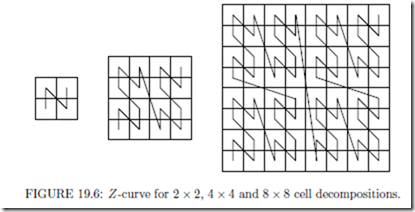Cell Orderings and Space-Filling Curves

We explore a suitable one dimensional ordering of cells and use it in conjunction with spatial ordering to develop eﬃcient algorithms for compressed quad trees. First, deﬁne an ordering for the immediate subcells of a cell. In two dimensions, we use the order SW, NW, SE and NE. The same ordering has been used to order the children of a node in a two dimensional

quadtree (Figure 19.2 and Figure 19.5).

Now consider ordering two arbitrary cells. If one of the cells is contained in the other, the subcell precedes the supercell. If the two cells are disjoint, the smallest supercell enclosing both the cells contains them in diﬀerent immediate subcells of it. Order the cells according to the order of the immediate subcells containing them. This deﬁnes a total order on any collection of cells with a common root cell. It follows that the order of leaf regions in a quad tree corresponds to the left-or-right order in which the regions appear in our drawing scheme. Similarly, the ordering of all regions in a quad tree corresponds to the postorder traversal of the quad tree. These concepts naturally extend to higher dimensions. Note that any ordering of the immediate subcells of a cell can be used as foundation for cell orderings.

Ordering of cells at a particular resolution in the manner described above can be related to space ﬁlling curves. Space ﬁlling curves are proximity preserving mappings from a multi- dimensional uniform cell decomposition to a one dimensional ordering. The path implied in the multidimensional space by the linear ordering, i.e., the sequence in which the multidimensional cells are visited according to the linear ordering, forms a non-intersecting curve. Of particular interest is Morton ordering, also known as the Z-space ﬁlling curve . The Z-curves for 2 × 2, 4 × 4 and 8 × 8 cell decompositions are shown in Figure 19.6. Consider a square two dimensional region and its 2k × 2k cell decomposition. The curve is considered to originate in the lower left corner and terminate in the upper right corner. The curve for a 2k × 2k grid is composed of four 2k−1 × 2k−1 grid curves one in each quadrant of the 2k × 2k grid and the tail of one curve is connected to the head of the next as shown in the ﬁgure. The order in which the curves are connected is the same as the order of traversal of the 2 × 2 curve. Note that Morton ordering of cells is consistent with the cell ordering speciﬁed above. Other space ﬁlling curves orderings such as graycode  and Hilbert  curve can be used and quad tree ordering schemes consistent with these can also be utilized. We will continue to utilize the Z-curve ordering as it permits a simpler bit interleaving scheme which will be presented and exploited later.

Algorithms on compressed quad tree rely on the following operation due to Clarkson :

LEMMA 19.1 Let R be the product of d intervals I1 × I2 × ... × Id, i.e., R is a hyper-rectangular region in d dimensional space. The smallest cell containing R can be found in O(d) time, which is constant for any ﬁxed d.

The procedure for computing the smallest cell uses ﬂoor, logarithm and bitwise exclusive- or operations. An extended RAM model is assumed in which these are considered constant time operations. The reader interested in proof of Lemma 19.1 is referred to [1, 4]. The operation is useful in several ways. For example, the order in which two points appear in the quad tree as per our ordering scheme is independent of the location of other points. To determine the order for two points, say (x1, x2,…, xd) and (y1, y2,…, yd), ﬁnd the smallest cell that contains [x1, y1] × [x2, y2] × ... × [xd, yd]. The points can then be ordered according to its immediate subcells that contain the respective points. Similarly, the smallest cell containing a pair of other cells, or a point and a cell, can be determined in O(d) time.

A Divide-and-Conquer Construction Algorithm

Let T1 and T2 be two compressed quad trees representing two distinct sets S1 and S2 of points. Let r1 (respectively, r2) be the root node of T1 (respectively, T2). Suppose that L(r1) = L(r2), i.e., both T1 and T2 are constructed starting from a cell large enough to

contain S1 S2. A compressed quad tree T for S1 S2 can be constructed in O(|S1| + |S2|) time by merging T1 and T2.

To merge T1 and T2, start at their roots and merge the two trees recursively. Suppose that at some stage during the execution of the algorithm, node u in T1 and node v in T2 are being considered. An invariant of the merging algorithm is that L(u) and L(v) cannot be disjoint. Furthermore, it can be asserted that S(u) S(v) L(u) L(v). In merging two nodes, only the small cell information is relevant because the rest of the large cell (L(v) S(v)) is empty. For convenience, assume that a node may be empty. If a node has

less than 2d children, we may assume empty nodes in place of the absent children. Four distinct cases arise: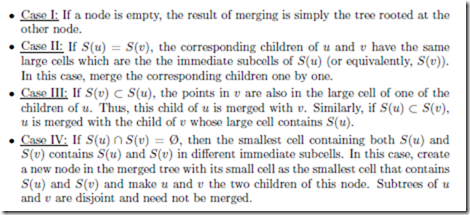LEMMA 19.2 Two compressed quad trees with a common root cell can be merged in time proportional to the sum of their sizes.

Proof The merging algorithm presented above performs a preorder traversal of each compressed quad tree. The whole tree may not need to be traversed because in merging a node, it may be determined that the whole sub tree under the node directly becomes a sub tree of the resulting tree. In every step of the merging algorithm, we advance on one of the trees after performing at most O(d) work. Thus, the run time is proportional to the sum of the sizes of the trees to be merged.

To construct a compressed quad tree for n points, scan the points and ﬁnd the smallest and largest coordinate along each dimension. Find a region that contains all the points and use this as the root cell of every compressed quad tree constructed in the process. Recursively construct compressed quadtrees for l n J points and the remaining p n l points and merge them in O(dn) time. The compressed quad tree for a single point is a single node v with the root cell as L(v). The run time satisﬁes the recurrence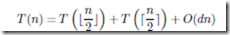resulting in O(dn log n) run time.

Bottom-up Construction

To perform a bottom-up construction, ﬁrst compute the order of the points in O(dn log n) time using any optimal sorting algorithm and the ordering scheme described previously. The compressed quadtree is then incrementally constructed starting from the single node tree for the ﬁrst point and inserting the remaining points as per the sorted list. During the insertion process, keep track of the most recently inserted leaf. Let p be the next point to be inserted. Starting from the most recently inserted leaf, traverse the path from the leaf

to the root until the ﬁrst node v such that p L(v) is encountered. Two possibilities arise:

CaseI: If p / S(v), then p is in the region L(v) S(v), which was empty previously. The smallest cell containing p and S(v) is a subcell of L(v) and contains p and S(v) in diﬀerent immediate subcells. Create a new node u between v and its parent and insert p as a child of u.

CaseII: If p S(v), v is not a leaf node. The compressed quadtree presently does not contain a node that corresponds to the immediate subcell of S(v) that contains p, i.e., this immediate subcell does not contain any of the points previously inserted. Therefore, it is enough to insert p as a child of v corresponding to this subcell.

Once the points are sorted, the rest of the algorithm is identical to a post-order walk on the ﬁnal compressed quad tree with O(d) work per node. The number of nodes visited per insertion is not bounded by a constant but the number of nodes visited over all insertions is O(n), giving O(dn) run time. Combined with the initial sorting of the points, the tree can be constructed in O(dn log n) time.

Basic Operations

Fast algorithms for operations on quad trees can be designed by simultaneously keeping track of spatial ordering and one dimensional ordering of cells in the compressed quad tree. The spatial ordering is given by the compressed quad tree itself. In addition, a balanced binary search tree (BBST) is maintained on the large cells of the nodes to enable fast cell searches. Both the trees consist of the same nodes and this can be achieved by allowing

each node to have pointers corresponding to compressed quad tree structure and pointers corresponding to BBST structure.

Point and Cell Queries

Point and cell queries are similar since a point can be considered to be a zero length cell. A node v is considered to represent cell C if S(v) C L(v). The node in the compressed

quad tree representing the given cell is located using the BBST. Traverse the path in the BBST from the root to the node that is being searched in the following manner: To decide which child to visit next on the path, compare the query cell with the large and small cells at the node. If the query cell precedes the small cell in cell ordering, continue the search with the left child. If it succeeds the large cell in cell ordering, continue with the right child. If it lies between the small cell and large cell in cell ordering, the node represents the query cell. As the height of a BBST is O(log n), the time taken for a point or cell query is O(d log n).

Insertions and Deletions

As points can be treated as cells of zero length, insertion and deletion algorithms will be discussed in the context of cells. These operations are meaningful only if a cell is inserted as a leaf node or deleted if it is a leaf node. Note that a cell cannot be deleted unless all its subcells are previously deleted from the compressed quad tree.

Cell Insertion

To insert a given cell C, ﬁrst check whether it is represented in the compressed quad tree. If not, it should be inserted as a leaf node. Create a node v with S(v) = C and ﬁrst insert v in the BBST using a standard binary search tree insertion algorithm. To insert v in the compressed quad tree, ﬁrst ﬁnd the BBST successor of v, say u. Find the smallest cell D containing C and the S(u). Search for cell D in the BBST and identify the corresponding node w. If w is not a leaf, insert v as a child of w in compressed quad tree. If w is a leaf, create a new node wl such that S(wl) = D. Nodes w and v become the children of wl in the compressed quad tree. The new node wl should be inserted in the BBST. The overall algorithm requires a constant number of insertions and searches in the BBST, and takes O(d log n) time.

Cell Deletion

As in insertion, the cell should be deleted from the BBST and the compressed quad tree. To delete the cell from BBST, the standard deletion algorithm is used. During the execution of this algorithm, the node representing the cell is found. The node is deleted from the BBST only if it is present as a leaf node in the compressed quad tree. If the removal of this node from the compressed quad tree leaves its parent with only one child, the parent is deleted as well. Since each internal node has at least two children, the delete operation cannot propagate to higher levels in the compressed quad tree.

Practical Considerations

In most practical applications, the height of a region quad tree is rather small because the spatial resolution provided by a quad tree is exponential in its height. This can be used to design schemes that will greatly simplify and speedup quad tree algorithms.

Consider numbering the 22k cells of a 2k × 2k two dimensional cell decomposition in the order speciﬁed by the Z-curve using integers 0 ... 4k 1 (Figure 19.6).

Represent each

cell in the cell space using a coordinate system with k bits for each coordinate. From the deﬁnition of the Z-curve, it follows that the number of a cell can be obtained by interleaving the bits representing the x and y coordinates of the cell, starting from the x coordinate.

For example, (3, 5) = (011, 101) translates to 011011 = 27. The procedure can be extended to higher dimensions. If (x1, x2,... xd ) represents the location of a d dimensional cell, the corresponding number can be obtained by taking bit representations of x1, x2,... xd, and interleaving them.

The same procedure can be described using uncompressed region quad trees. Label the 2d edges connecting a node to its children with bit strings of length d. This is equivalent to describing the immediate subcells of a cell using one bit for each coordinate followed by bit interleaving. A cell can then be described using concatenation of the bits along the path from the root to the cell. This mechanism can be used to simultaneously describe cells at various length scales. The bit strings are conveniently stored in groups of 32 or 64 bits using integer data types. This creates a problem in distinguishing certain cells. For instance, consider distinguishing cell “000” from cell “000000” in an octree. To ensure each bit string translates to a unique integer when interpreted as a binary number, it is preﬁxed by a 1. It also helps in easy determination of the length of a bit string by locating the position of the leftmost 1. This leads to a representation that requires dk + 1 bits for a d dimensional quad tree with a height of at most k. Such a representation is very beneﬁcial because primitive operations on cells can be implemented using bit operations such as and, or, exclusive-or etc. For example, 128 bits (4 integers on a 32 bit computer and 2 integers on a 64 bit computer) are suﬃcient to describe an octree of height 42, which

allows representation of length scales 242 : 1 > 4 × 1012 : 1.

The bit string based cell representation greatly simpliﬁes primitive cell operations. In the following, the name of a cell is also used to refer to its bit representation:

Check if C1 C2. If C2 is a preﬁx of C1, then C1 is contained in C2, otherwise not.

Find the smallest cell enclosing C1 and C2. This is obtained by ﬁnding the longest common preﬁx of C1 and C2 whose length is 1 mod d.

Find the immediate subcell of C1 that contains C2. If dl + 1 is the number of bits representing C1, the required immediate subcell is given by the ﬁrst (d + 1)l + 1 bits of C2.

Consider n points in a root cell and let k denote the largest resolution to be used. Cells are not subdivided further even if they contain multiple points. From the coordinates of a point, it is easy to compute the leaf cell containing it. Because of the encoding scheme used, if cell C should precede cell D in the cell ordering, the number corresponding to the binary interpretation of the bit string representation of C is smaller than the corresponding number for D. Thus, cells can be sorted by simply treating them as numbers and ordering them accordingly.

Finally, binary search trees and the attendant operations on them can be completely avoided by using hashing to directly access a cell. An n leaf compressed quad tree has at most 2n 1 nodes. Hence, an array of that size can be conveniently used for hashing. If all cells at the highest resolution are contained in the quad tree, i.e., n = dk , then an array of size 2n 1 can be used to directly index cells. Further details of such representation are left as an exercise to the reader.